## Abstract

The value of the gas-path parameter, exhaust gas temperature margin (EGTM), is the critical index for predicting aeroengine performance degradation. Accurate predictions help to improve engine maintenance, replacement schedules, and flight safety. The outside air temperature (OAT), altitude of the airport, the number of flight cycles, and water washing information were chosen as the sample input variables for the data-driven prognostic model for predicting the take-off EGTM of the on-wing engine. An attention-based deep learning framework was proposed for the aeroengine performance prediction model. Specifically, the multiscale convolutional neural network (CNN) structure is designed to initially learn sequential features from raw input data. Subsequently, the long short-term memory (LSTM) structure is employed to further extract the features processed by the multiscale CNN structure. Furthermore, the proposed attention mechanism is adopted to learn the influence of features and time steps, assigning different weights according to their importance. The actual operation data of the aeroengine are used to conduct experiments, where the experimental results verify the effectiveness of our proposed method in EGTM prediction.

Keywords: Convolution neural network, long short-term memory, attention mechanism, aeroengine gas-path performance, exhaust gas temperature margin

## 1. Introduction

The aeroengine operates at the highest temperature, pressure, speed, and frequency of transitional working states during the take-off phase when compared to the other flight phases like cruise and landing [1,2]. The slow decline of engine performance is inevitable during the active process. Prediction and evaluation of the decline degree of engine performance are necessary for performing preventive maintenance on the aeroengine. Most engine failures are caused by the gas-path system fault, and accurate gas-path performance prediction provides the possibility for aeroengine performance evaluation and maintenance plan optimization. This is significant for ensuring the flight safety of aircraft.

The gas path parameters include exhaust gas temperature (EGT), rotor speed, and fuel flow. The EGT margin (EGTM) is usually adopted to perform gas path analysis and monitor the engine performance degradation, which can show whether the aeroengines are in the normal state or not . During actual monitoring and maintenance, the take-off EGTM  is usually chosen as the critical gas-path parameter to evaluate the performance state of the aeroengine. A deteriorated engine will consume more fuel, thus increasing the EGT and decreasing the EGTM . The net thrust, fuel flow, low rotor speed, core rotor speed, pressure ratio, air temperature at engine fan inlet, take-off EGTM, and specific fuel consumption are regarded as input parameters for estimating the EGT . These input parameters were collected by the sensors during the flight , and they are unknown for the prognostic analysis. In that work, the relationship between EGT decline rate and the flight cycles was established to predict the remaining life of a PW4000-94 engine. The EGTM was influenced by the unknown real-time data, obtained by sensor data during the flight, as well as the known data obtained before the flight. This work aims to utilize the above-known data as the input parameters to predict the EGTM prior to flight.

Take-off EGTM, as shown in Figure 1, reflects the state of engine performance. When the take-off EGTM equals 0 °C, the EGT has reached the red line value. The EGTM in the take-off phase directly relates to the airport outside air temperature (OAT) and altitude. However, empirical data do not exist to allow a correlation between EGTM deterioration and the OAT or altitude of the airport.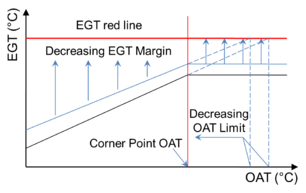Figure 1. Effect of OAT in the airport on EGTM deterioration

The value of EGTM significantly affects engine life. Reducing EGTM will extend engine life on the wing, thereby reducing operating costs. If the engine is arranged to take off at the airport at a lower OAT and altitude, EGT will likely not cross the red line. With regard to meeting the aircraft performance requirements, the engine is designed to provide a given thrust level at a temperature below the corner point OAT. As the OAT in the airport increases, more fuel is required, EGT increases, and EGTM decreases. However, at a temperature above the corner point OAT, the EGTM is less than zero, and the thrust output must be reduced. If it does not reduce, the engine will be damaged . Similarly, as the altitude of the airport increases, more fuel will be required to provide a given thrust output, so the EGT will increase, resulting in a decrease in the EGTM .

The number of flight cycles significantly affects the take-off EGTM of aeroengines. For an available gas turbine engine, the levels of degradation drop by increasing the flight cycles, and all engine health parameters deviate slowly from their nominal values . The degradation data of the booster were used to illustrate the clean compressor map and degraded compressor maps at 3000 and 6000 flight cycles for the JT9D turbofan engine . The degraded maps were utilized to predict the overall degradation effects on the engine performance. In the take-off phase, the engine accelerates from idle to maximum power resulting in maximum rotor speed and EGT, which causes the turbine blade to elongate and creep . In addition, abrasion between the elongated turbine blade and the stationary parts occurs. The turbine clearance increases during the thermal cycles, such as the start and stop cycles, namely flight cycles. The engine wear and higher clearance lead to a deterioration of the engine efficiency, which decreases the turbine efficiency . In this case, more fuel is consumed to maintain a given thrust level, so the EGTM will decrease, and the engine performance will degrade.

Meanwhile, low cycle fatigue (LCF)  is associated with engines that have been in service for long periods. LCF occurs due to machine cyclic loading, like start/stop cycles, which is closely related to the flight cycles. The LCF life of a component is determined by the number and the intensity of cycles the component material must endure . In contrast, the creep life of a component depends on the time it spends operating within the material's creep temperature range. The number of flight cycles can influence the communicative time effect on the wear, creep, and fatigue life of hot section components.

Periodical on-wing water washing is an efficient and economical method to improve engine performance and restore the take-off EGTM . Engines in the take-off phase and the approaching landing phase of each flight cycle are more affected by the airborne pollutant due to the lower altitude they operate, making them more susceptible to compressor fouling degradation. The changing value of the mass flow rate and the compressor's efficiency due to compressor fouling can be expressed as a linear relationship concerning the flight cycle . Besides the effect of online washing with different water-to-air ratios and engine loads on performance recovery , the effect of inlet pressure and droplet diameter of washing liquid on compressor fouling removal , and the recovery efficiency of power loss with washing  have been studied.

To predict the take-off EGTM of the on-wing engine in advance, we choose the OAT and altitude of the airport, the number of flight cycles, and water washing information as the sample input variables for a prediction algorithm. All these parameters can be obtained before the flight, making the prognostic of the engine's performance degradation known in advance. The aeroengine performance degradation prediction is a time series forecasting task. Deep architectures such as convolutional neural networks (CNN) and long short-term memory (LSTM) can extract and effectively capture the feature information of raw input data. However, the ability of normal CNN is affected by the size of convolution kernels, which should be accurately determined. Another key issue is that once the sequence is too long, the traditional LSTM cannot effectively use the location information of time series data and capture the long-term interdependence. As such, an attention mechanism was added for assigning different weights to features of different importance.

The main contributions of this paper are summarized as follows:

1) A multiscale CNN-LSTM structure is developed to handle raw aeroengine data to learn temporal sequence features and extract useful degradation information.
2) We propose a deep learning framework based on an attention mechanism. The attention mechanism can learn the importance of sequential features and time steps, assigning different weights according to their preference.
3) We conduct experiments on real datasets to evaluate the effectiveness of our proposed method. The experimental results show that the proposed method shows a considerable improvement in aeroengine performance estimation.

The rest of this paper is organized as follows. Section 2 reviews the related works. Section 3 describes the suggested method based on deep learning, and section 4 includes the computational experiments and an analysis of the results. Finally, section 5 concludes this work and gives future studies.

## 2. Related works

Multiple methods are utilized in engineering applications to analyze the gas-path system for aeroengine performance prediction. In general, these prediction methods can be divided into two categories: model-based methods – and data-driven methods . The nonlinear simulation model of a twin-spool turbofan engine was constructed as a component level model by Adibhatla et al. . A bank of parallel Kalman filters and a hierarchical structure were used for the multiple model adaptive estimation methods of in-flight failures test by Maybeck . The nonlinear dynamics of the jet engine are linearized and a set of linear models corresponding to various operating modes of the engine at each operating point is obtained by Lu et al . However, the component structures and accessory systems of aeroengines are becoming increasingly complex and integrated. Accurate modeling remains difficult with model-based methods due to the challenge of mastering various nonlinear mathematical relationships between components and systems .

When compared with these model-based methods, data-driven methods do not require an understanding of the complex operation mechanisms of the mechanical system. Therefore, data-driven methods have been widely used in aeroengine performance prediction. The previously collected data of gas path systems were time-series data of multiple state parameters. A CNN is designed to extract the features from this input data. The CNN prediction technology was combined with a delta fuel flow degradation baseline to estimate the performance recovery by the water washing . A CNN-based multitask learning framework was proposed to accurately estimate the remaining useful life (RUL) by simultaneous learning. The estimations occurred during a health state identification, where inter-dependencies of both tasks were considered using general features extracted from the shared network . A CNN and extreme gradient boosting (CNN-XGB) were combined through model averaging. A CNN-XGB with an extended time window was utilized for a RUL estimation .

A hybrid method of convolutional and recurrent neural network (CNN-RNN) was proposed for the RUL estimation, where it can extract the local features and capture the degradation process . A RNN has the advantage of a data-driven model with short time dependencies. Nevertheless, a RNN has poor performance in dealing with long-time dependencies data. LSTM neural networks have been proposed to address these dependencies for predicting the RUL of any system. The LSTM model has the advantage of retaining time domain information for a long duration of time. The accuracy of an online LSTM method was improved by comparing it to the proposed methods in Kakati et al.  for RUL estimation of a turbofan engine. The LSTM, as well as the statistical process analysis, were performed to predict the fault of aeroengine components with multi-stage performance degradation . The linear regression model and LSTM were utilized to construct the data-driven model of degradation trend prediction and RUL estimation . RUL estimation for predictive maintenance was achieved by using the support vector regression (SVR) model and an LSTM network .

A novel performance degradation prediction method based on the attention model and SVR is proposed for RUL prediction. The attention mechanism can focus on the important features in the time-sequential data, while the SVR model identifies the mapping relationship between multiple state parameters and performance degradation . Many hidden layers were constructed for the machine learning model and a large number of training data to learn more useful features and improve the accuracy of classification and prediction. The designed system  is based on reinforcement learning and a deep learning framework, which consists of an input, modeling, and a decision layer. Li et al.  proposed a new data-driven approach for prognostics by using deep CNN. In that work, a time window approach employed for sample preparation achieves better feature extraction by deep CNN leading to high prognostic accuracy with regards to the RUL estimation.

An intelligent deep learning method was proposed for forecasting the health evolution trend of aeroengine by Jiang et al. . This method systematically blends the dispersion entropy-based multi-scale series aggregation scheme with a long LSTM neural network. Remadna et al.  introduced a new hybrid RUL prediction approach by combining two deep learning methods sequentially. The hybrid model uses a CNN with bidirectional LSTM networks where the CNN extracts spatial features while bidirectional LSTM extracts temporal features. Chu et al.  proposed an integrated deep learning approach with CNN and LSTM networks to learn the latent features and estimate RUL value with a deep survival model based on the discrete Weibull distribution. In their work, the turbofan engine degradation simulation datasets provided by NASA were utilized to validate the proposed approach.

## 3. Methodology

This section describes the aeroengine EGTM prediction problem. Next, the proposed attention-based multiscale CNN-LSTM method is introduced in detail. This includes the theoretical background of the components and the method’s overall framework.

### 3.1 Problem description

From the perspective of health management, aeroengine EGTM prediction can be regarded as a time series problem. The EGTM prediction problem can be defined as follows. The input is ${\textstyle {X}_{t}^{k}}$, where ${\textstyle k\in R^{n}}$, ${\textstyle t=(1,2,\ldots ,T)}$. In addition, ${\textstyle {X}_{t}^{k}}$ represents the collected input data during aeroengine operation, $n$ represents the number of features, and $T$ represents the length of the time step. The corresponding output is the EGTM prediction result ${\textstyle {Y}_{t}}$ for each time step. EGTM is predicted in real-time by establishing the mapping relationship between the output and input data. The mapping relationship is expressed as follows:

 ${Y}_{t}=f({X}_{t}^{k})$
(1)

When building the performance prediction model, the above input data are directly imported from the raw data file to predict EGTM. As such, a large amount of mixed noise exists in the data. To fully extract the time-series features of the data, we design a multiscale CNN-LSTM deep learning framework based on an attention mechanism to construct mapping relationships, as introduced in detail in the following subsections.

### 3.2 Multiscale CNN

A traditional CNN can directly process the input raw data and extract the hidden features. However, the amount of raw data is relatively large. Thus, using a single convolution kernel may cause the model to omit locally important features in the process of adaptively extracting features. By adjusting the scale of the convolution kernel and using several different convolution kernels, designing a network capable of extracting the raw data features may be possible. This results in the performance of the model prediction improving.

This work proposes an improved CNN with a multiscale convolution operation to compensate for the limitation of a traditional CNN. Specifically, each convolution layer consists of 64 convolution kernels, and we set the convolution kernel size to 1, 3, and 5. The multiscale convolution operation is embodied as a structure to extract the hidden features by performing a multiscale convolution operation on the raw data. Initially, this establishes a shallow mapping relationship between the raw data and EGTM. The specific network structure is shown in Figure 2.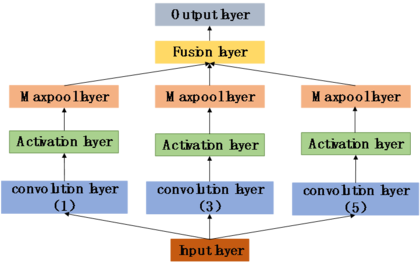Figure 2. Structure of multiscale CNN

### 3.3 Long short-term memory network

The data in the proposed prediction model, discussed previously, are time series, the nodes of the RNN are connected along the sequence, and the RNN is designed to learn the correlation of the time series. However, the standard RNN often encounters the problem of gradient disappearance and gradient explosion during the training process. As a result, both the model’s ability to capture the previous information and its performance in modeling long-term dependencies decreases.

To solve this problem, Hochreiter proposed a new architecture named long and short-term memory network (LSTM) . LSTM is a special RNN, which has been widely used in various time sequence modeling tasks such as stock market price prediction and energy consumption prediction. The advantage of LSTM involves its ability to overcome shortcomings of traditional RNN, such as the influence of gradient disappearance and gradient explosion. The basic architecture of a typical LSTM is shown in Figure 3.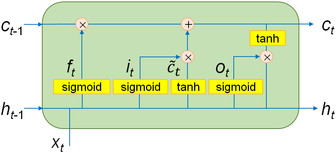Figure 3. Structure of LSTM

One notable feature includes how it delicately designs the structure of the recurrent unit. The sigmoid activation function, tanh activation function, and element-wise product work together to form three gate structures: forget gate, input gate, and output gate. Two gates are used to control the state of the memory cell ${\textstyle c}$. The first gate is the forget gate, while the other is the input gate. When the forget gate is turned on, some information from the previous memory cell state ${\textstyle c_{t-1}}$ could be ignored, and others will be kept. When the input gate is activating, the information from the current input ${\textstyle x_{t}}$ can be added to the memory cell ${\textstyle c}$. LSTM uses the output gate to control how much information of the memory cell state ${\textstyle c_{t}}$ will be added to the current output ${\textstyle h_{t}}$. For the given inputs ${\textstyle x_{t}}$, ${\textstyle h_{t-1}}$, and ${\textstyle c_{t-1}}$, the update process of LSTM for time step ${\textstyle t}$ is shown as Eq.(2)

 $\left\{{\begin{matrix}\&{i}_{t}=\sigma ({W}_{i}[{h}_{t-1},{x}_{t}]+{b}_{i})\\\&{f}_{t}=\sigma ({W}_{f}[{h}_{t-1},{x}_{t}]+{b}_{f})\\\&{o}_{t}=\sigma ({W}_{o}[{h}_{t-1},{x}_{t}]+{b}_{o})\\\&{\tilde {c}}_{t}=\tanh({W}_{c}[{h}_{t-1},{x}_{t}]+{b}_{c})\\\&{c}_{t}={f}_{t}\odot {c}_{t-1}+{i}_{t}\odot {\tilde {c}}_{t}\\\&{h}_{t}={o}_{t}\odot \tanh({c}_{t})\end{matrix}}\right.$
(2)

In the above equation, ${W}_{i}$, ${W}_{f}$, ${W}_{o}$, and ${W}_{c}$ are the weight matrices for connections, $b_{i}$, $b_{f}$, $b_{o}$ and $b_{c}$ are the bias vectors，and $\sigma (\cdot )$ and tanh are the sigmoid and $\tanh$ functions, respectively. As mentioned above, LSTM has played an essential role in various tasks required to model time series data, which demonstrates the effectiveness of LSTM in addressing time series prediction problems. However, the regression of the standard LSTM is often based on the features learned in the last time step. It cannot accurately control the sequence impact of each time on the output, leading to a decrease in the final prediction accuracy. Hence, we added an attention mechanism to the proposed framework for learning the importance of each time step. So the neural network can learn and extract helpful feature information more thoroughly.

### 3.4 Attention mechanism

In recent years, an attention mechanism has been widely used in various tasks of deep learning, such as image caption generation , speech recognition , and visual question answering . An attention mechanism, inspired by the ability of humans to focus on specific information while ignoring others selectively, can make deep learning more targeted when extracting the features for improving the accuracy of related prediction tasks. In addition, this operation does not increase the cost of model calculation or storage.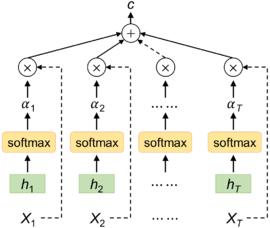Figure 4. Structure of the attention mechanism

Applying the attention mechanism shown in Figure 4 to the EGTM prediction is achieved by assigning different weights to different features for focusing on the regions of different importance. Adding the weights into the neural network is useful for distinguishing various features. The first step of the specific process defines the input of the attention layer as the learned feature state ${\textstyle H=(h_{1},h_{2},.....,h_{T})}$. This is activated by the LSTM layer, where the calculation formula of the score ${\textstyle S_{t}}$ of the $t$-th feature is expressed by the following equation

 ${S}_{t}=\tanh \left({W}_{h}{h}_{t}+b\right)$
(3)

In Eq.(3), ${W}_{h}$ and $b$ represent the weight matrix and the bias vector, respectively, while tanh serves as an activation function. After the score ${\textstyle S_{t}}$ is obtained, it is normalized by the softmax function

 ${a}_{t}={\frac {\exp \,(S_{t})}\sum _{t=1}^{T}\exp \,({S}_{t})}}$
(4)

The final output feature $c$ of the attention mechanism can be expressed as:

 $c=\sum _{t=1}^{T}{a}_{t}{h}_{t}$
(5)

### 3.5 Overall framework

Figure 5 shows the overall framework of our proposed method for EGTM prediction. It is a multiscale CNN-LSTM model based on a multiscale convolution kernel and an attention mechanism. The general framework comprises three substructures: a CNN layer (including multiscale convolution layer, pooling layer, and feature fusion layer), a LSTM layer, and an attention layer.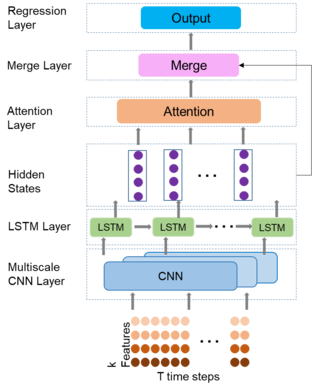Figure 5. Structure of the overall framework

The operation process of the proposed model starts with feature extraction performed on the raw collected data of aeroengines. The process utilizes a multiscale CNN structure to perform convolution operations for extracting representative features. The CNN consists of three multiple convolution layers of different scales, the maxpool layer, and the fusion layer. Next, the method employs the LSTM structure for further feature learning to find trends within the data. Then, the features processed by LSTM are transferred to the attention layer. Using the attention mechanism to learn the entire sequence simultaneously, the position information of the features can be considered with weights generated to inform the neural network with regards to extracting useful detailed information for improving the performance of the aeroengine EGTM prediction. Subsequently, the features learned by LSTM are merged with the importance weights generated by the attention mechanism. Finally, the regression layer is used to output the results of EGTM prediction. The inputs of the key modules (the multiscale CNN layer, the LSTM layer, the attention layer, and the merged layer) are summarized in Table 1.

Table 1. Inputs of each key layer
Layer Input
Multiscale CNN (MCNN) Raw aeroengine data
LSTM Features learned by MCNN
Attention mechanism Features learned by LSTM
Merge layer Features learned by LSTM weights generated by the attention mechanism

## 4. Experiments

### 4.1 Experimental datasets

To verify the performance of the proposed method, the EGTM data are collected from a civil aviation turbofan engine. The altitude data from the airport and flight cycles are obtained from historical flight records and a future flight plan. The OAT data in the airport are inferred from historical flight records and a future flight plan . The historical atmosphere information of the airports and the water washing information can be collected from engine maintenance records.

### 4.2 Data preprocessing

#### 4.2.1 Normalization

Data from different sources have various units and scales, which could affect the accuracy of EGTM prediction. Therefore, the raw input is normalized to speed up training convergence and improve the generalization ability. This paper adopts the min-max normalization method to preprocess data. In general, the raw input data is mapped to the interval 0~1. Specifically, for the input data ${\textstyle {X}_{t}^{k}}$, we normalize it as follows:

 ${\tilde {{X}_{t}^{k}}}={\frac {{X}_{t}^{k}-\min \,\left\{{X}^{k}\right\}}{\max \,\left\{{X}^{k}\right\}-\min \,\left\{{X}^{k}\right\}}}$
(6)

where ${\textstyle {\tilde {{X}_{t}^{k}}}}$ represents the normalized data, ${\textstyle \max \,\left\{{X}^{k}\right\}}$ and ${\textstyle \min \,\left\{{X}^{k}\right\}}$ represent the maximum and minimum values in the sequence, respectively.

#### 4.2.2 Sliding time-window processing

In problems based on multivariate time series, time series data sampled at a longer temporal sequence usually have more information than a data point with a single time step. A sliding window is used for data segmentation to use the multivariate temporal information and generate the network inputs. An example of data segmentation through sliding time window processing is shown in Figure 6. The window size is denoted as ${\textstyle s}$, and the sliding step is set to be expressed as ${\textstyle l}$. The short sliding step can increase the number of experimental samples to reduce the risk of overfitting and ensure the stability of the training process. As such, the sliding step is set to a value of 1.0. We will discuss the impact of time window size on the model prediction performance in Section 4.5.1.Figure 6. Sliding time window processing

### 4.3 Evaluation criteria

To evaluate the performance of the RUL prediction, we use two commonly adopted evaluation criteria, root mean square error (RMSE) and mean absolute error (MAE). RMSE and MAE are defined as follows by Eqs.(7) and (8), respectively:

 $RMSE={\sqrt {{\frac {1}{N}}\sum _{i=1}^{N}({R}_{i}-{P}_{i}{)}^{2}}}$
(7)
 $MAE={\frac {1}{N}}\sum _{i=1}^{N}\left|{R}_{i}-{P}_{i}\right|$
(8)

In Eqs.(7) and (8), ${\textstyle N}$ represents the number of testing samples, and ${\textstyle R_{i}}$ and ${\textstyle P_{i}}$ represent the actual EGTM and predicted EGTM of the ${\textstyle i}$-th sample, respectively.

### 4.4 Structural parameters

The number of the hidden units and the size of the convolutional kernel in each layer are set to the same value to simplify the parameter selection. The mini-batch gradient descent method is used to train the network, and the batch size is set to 8. The Adam algorithm has the advantages of the back-propagation algorithm and possesses an excellent ability with handling non-stationary data, so the Adam algorithm is adopted to train the neural networks.

The structural parameters of attention-based multiscale CNN-LSTM are determined by contrast experiments. The parameters include the output dimension of CNN, the number of stacked layers of LSTM, and the dimension of hidden layers. They are determined this way obtain a better prediction performance. When deciding the output dimensions of CNN by contrast experiments, the output dimensions are used as variables, while other structural parameters are used as definite values, simultaneously. Similarly, the number of stacked layers and hidden layer dimensions of LSTM are also determined through comparative experiments. Table 2 lists the final parameters of each part of the structure.

Table 2. Structural Parameters of our method
Layers Parameters
Hidden units: 64 Pool: Maxpooling1D
Activation: Relu
LSTM Hidden units: 128
Num_layer: 4
Dropout: 0.2
Attention Dense:128
Activation: Relu
Dense: 1

### 4.5 Experimental results and analysis

To verify the effectiveness of the proposed method, we first analyze the influence of window size on the prediction performance of EGTM. Then, ablation experiments are conducted to establish the role of the proposed multiscale CNN architecture and attention mechanism in improving the model's accuracy.

During the data processing, mentioned above, the selection of the time window size determines the input data of the network. Different time series data contain various information, so a reasonable time window size must be chosen. To evaluate the impact of the time window size, we conducted experiments to analyze the influence of window size on the prediction performance of EGTM. The results with different window sizes (i.e., 2, 3, 4, 5, 6, 7, 8, 9, 10, 11, and 12) are plotted in Figure 7. The figure shows fluctuations in the model's performance, but the overall trend indicates the performance improves when the window size is first increased. This may be due to the EGTM prediction containing more sequence information. When the window size is set to 4, the RMSE value and MAE reach a minimum. Increasing the window size leads to decreased model performance. Therefore, as the optimal size, the length of time window size is set to 4.

 Figure 7. Effect of the time window size on the prognostic performance

Furthermore, ablation experiments are conducted for the proposed method to evaluate the effectiveness of the proposed multiscale CNN structure and attention mechanism in improving the prediction accuracy of EGTM. Specifically, we conducted experiments on a traditional CNN-LSTM, a traditional CNN-LSTM with an attention mechanism, a multiscale CNN-LSTM, and our proposed method.

For training, we set the epoch to 300 and the mean square error (MSE) as the loss function. The parameters used in each model are identical. As shown in Figure 8, the red line indicates that the proposed method produces the smallest degree of error throughout the epochs.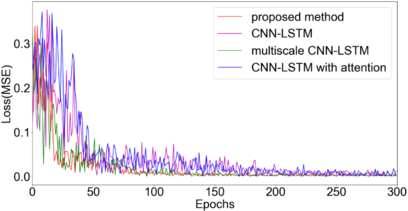Figure 8. Training loss of the proposed method and other models in ablation experiments

As shown in Figure 9, the performance of a multiscale CNN-LSTM and a traditional CNN-LSTM integrated with an attention mechanism performs better than a traditional CNN-LSTM. In addition, the proposed method in this paper has the highest accuracy in EGTM prediction when compared with other methods. This verifies the effectiveness of our proposed method in enhancing the performance of extracting data features from the input data.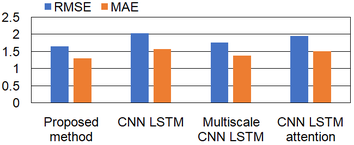Figure 9. Performance comparison of the proposed method and other models in ablation experiments

To analyze the predicted accuracy of our model with regard to EGTM, we compare the predicted EGTM with the actual EGTM of the aeroengine and plot the results in Figure 10. The trajectory of the predicted EGTM is similar to the real EGTM of the aeroengine when compared with other methods. This supports effectiveness of our proposed model in learning aeroengine degradation information. When compared with other stages, the error tends to be larger as EGTM is between 70 and 75, which may be a result of how the aeroengine begins to degenerate rapidly in this state.

 Figure 10. Analysis of EGTM prediction results of different models

## 5. Conclusion and future work

This paper proposes an attention-based multiscale CNN-LSTM framework for aeroengine performance prediction. First, a multiscale CNN is developed to extend the feature information of the raw input data to a longer time scale. Then, LSTM is used to learn the time-series features. An attention mechanism is adopted to obtain the importance of the time series data and assign different importance weights to the features for improving the prediction accuracy. Experiments on real data sets verify the effectiveness of the designed method in aeroengine performance prediction.

In prognostics and health management (PHM), accurate prediction performance is significant for ensuring the reliability of aeroengines and making maintenance plans and flight schedules. The price of data collection for the whole life of an aeroengine is relatively high, as it may take a long period of several years to track the degradation process. However, there are individual differences among aeroengines due to different manufacturing, assembly, and even model types. As such, they likely work under various scenarios. Hence, data-driven methods perform worse than expected. To address this problem and meet the actual needs of airline management and maintenance, the knowledge of transfer learning can be used to enhance the generalization ability of a data-driven prognostic model , so the trained model can be applied to the performance prediction of other aeroengines.

## Funding

This research was funded by the Science and Technology Foundation of Sichuan, grant number 2022YFG0356, the Key Research and Development Plan Foundation of Tibet, grant number XZ202101ZY0017G, and the Aeroengine Operational Safety and Control Technology Research Center Project of CAFUC, grant number JG2019-02-03 and D202105.

Conflicts of Interest: The authors declare no conflict of interest.

### Document informationPublished on 16/05/23
Accepted on 08/05/23
Submitted on 08/05/22

Volume 39, Issue 2, 2023
DOI: 10.23967/j.rimni.2023.05.002

### Document Score1

Views 30
Recommendations 1